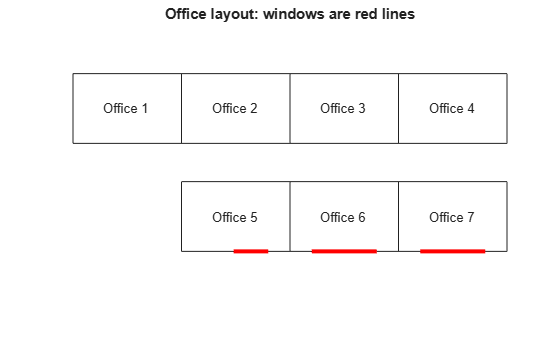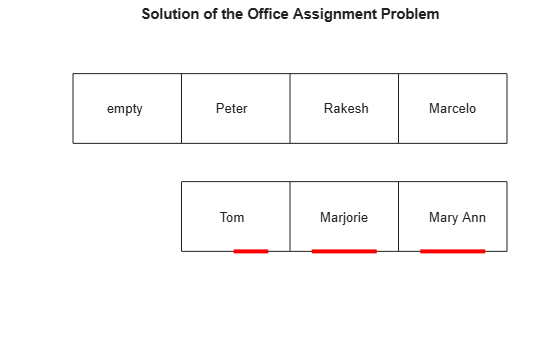Documentation

## Office Assignments by Binary Integer Programming: Solver-Based

This example shows how to solve an assignment problem by binary integer programming using the `intlinprog `function. For the problem-based approach to this problem, see Office Assignments by Binary Integer Programming: Problem-Based.

### Office Assignment Problem

You want to assign six people, Marcelo, Rakesh, Peter, Tom, Marjorie, and Mary Ann, to seven offices. Each office can have no more than one person, and each person gets exactly one office. So there will be one empty office. People can give preferences for the offices, and their preferences are considered based on their seniority. The longer they have been at the MathWorks, the higher the seniority. Some offices have windows, some do not, and one window is smaller than others. Additionally, Peter and Tom often work together, so should be in adjacent offices. Marcelo and Rakesh often work together, and should be in adjacent offices.

### Office Layout

Offices 1, 2, 3, and 4 are inside offices (no windows). Offices 5, 6, and 7 have windows, but the window in office 5 is smaller than the other two. Here is how the offices are arranged.

```name = {'Office 1','Office 2','Office 3','Office 4','Office 5','Office 6','Office 7'}; printofficeassign(name)```### Problem Formulation

You need to formulate the problem mathematically. First, choose what each element of your solution variable `x` represents in the problem. Since this is a binary integer problem, a good choice is that each element represents a person assigned to an office. If the person is assigned to the office, the variable has value 1. If the person is not assigned to the office, the variable has value 0. Number people as follows:

1. Mary Ann

2. Marjorie

3. Tom

4. Peter

5. Marcelo

6. Rakesh

`x` is a vector. The elements `x(1)` to `x(7)` correspond to Mary Ann being assigned to office 1, office 2, etc., to office 7. The next seven elements correspond to Marjorie being assigned to the seven offices, etc. In all, the `x` vector has 42 elements, since six people are assigned to seven offices.

### Seniority

You want to weight the preferences based on seniority so that the longer you have been at MathWorks, the more your preferences count. The seniority is as follows: Mary Ann 9 years, Marjorie 10 years, Tom 5 years, Peter 3 years, Marcelo 1.5 years, and Rakesh 2 years. Create a normalized weight vector based on seniority.

```seniority = [9 10 5 3 1.5 2]; weightvector = seniority/sum(seniority);```

### People's Office Preferences

Set up a preference matrix where the rows correspond to offices and the columns correspond to people. Ask each person to give values for each office so that the sum of all their choices, i.e., their column, sums to 100. A higher number means the person prefers the office. Each person's preferences are listed in a column vector.

```MaryAnn = [0; 0; 0; 0; 10; 40; 50]; Marjorie = [0; 0; 0; 0; 20; 40; 40]; Tom = [0; 0; 0; 0; 30; 40; 30]; Peter = [1; 3; 3; 3; 10; 40; 40]; Marcelo = [3; 4; 1; 2; 10; 40; 40]; Rakesh = [10; 10; 10; 10; 20; 20; 20];```

The ith element of a person's preference vector is how highly they value the ith office. Thus, the combined preference matrix is as follows.

`prefmatrix = [MaryAnn Marjorie Tom Peter Marcelo Rakesh];`

Weight the preferences matrix by `weightvector` to scale the columns by seniority. Also, it's more convenient to reshape this matrix as a vector in column order so that it corresponds to the `x` vector.

```PM = prefmatrix * diag(weightvector); c = PM(:);```

### Objective Function

The objective is to maximize the satisfaction of the preferences weighted by seniority. This is a linear objective function

`max c'*x`

or equivalently

`min -c'*x`.

### Constraints

The first set of constraints requires that each person gets exactly one office, that is for each person, the sum of the `x` values corresponding to that person is exactly one. For example, since Marjorie is the second person, this means that `sum(x(8:14))=1`. Represent these linear constraints in an equality matrix `Aeq` and vector `beq`, where `Aeq*x = beq`, by building the appropriate matrices. The matrix `Aeq` consists of ones and zeros. For example, the second row of `Aeq` will correspond to Marjorie getting one office, so the row looks like this:

`0 0 0 0 0 0 0 1 1 1 1 1 1 1 0 0 0 0 0 0 0 0 0 0 0 0 ... 0 0 0`

There are seven 1s in columns 8 through 14 and 0s elsewhere. Then `Aeq(2,:)*x = 1` is equivalent to `sum(x(8:14)) = 1`.

```numOffices = 7; numPeople = 6; numDim = numOffices * numPeople; onesvector = ones(1,numOffices);```

Each row of `Aeq` corresponds to one person.

```Aeq = blkdiag(onesvector,onesvector,onesvector,onesvector, ... onesvector,onesvector); beq = ones(numPeople,1);```

The second set of constraints are inequalities. These constraints specify that each office has no more than one person in it, i.e., each office has one person in it, or is empty. Build the matrix `A` and the vector `b` such that `A*x <= b` to capture these constraints. Each row of `A` and `b` corresponds to an office and so row 1 corresponds to people assigned to office 1. This time, the rows have this type of pattern, for row 1:

`1 0 0 0 0 0 0 1 0 0 0 0 0 0 1 0 0 0 0 0 0 ... 1 0 0 0 0 0 0`

Each row after this is similar but shifted (circularly) to the right by one spot. For example, row 3 corresponds to office 3 and says that `A(3,:)*x <= 1`, i.e., office 3 cannot have more than one person in it.

```A = repmat(eye(numOffices),1,numPeople); b = ones(numOffices,1);```

The next set of constraints are also inequalities, so add them to the matrix `A` and vector `b`, which already contain the inequalities from above. You want Tom and Peter no more than one office away from each other, and the same with Marcelo and Rakesh. First, build the distance matrix of the offices based on their physical locations and using approximate Manhattan distances. This is a symmetric matrix.

`D = zeros(numOffices);`

Set up the top right half of the matrix.

```D(1,2:end) = [1 2 3 2 3 4]; D(2,3:end) = [1 2 1 2 3]; D(3,4:end) = [1 2 1 2]; D(4,5:end) = [3 2 1]; D(5,6:end) = [1 2]; D(6,end) = 1;```

The lower left half is the same as the upper right.

`D = triu(D)' + D;`

Find the offices that are more than one distance unit away.

```[officeA,officeB] = find(D > 1); numPairs = length(officeA)```
```numPairs = 26 ```

This finds `numPairs` pairs of offices that are not adjacent. For these pairs, if Tom occupies one office in the pair, then Peter cannot occupy the other office in the pair. If he did, they would not be adjacent. The same is true for Marcelo and Rakesh. This gives `2*numPairs` more inequality constraints that you add to `A` and `b`.

Add enough rows to `A` to accommodate these constraints.

```numrows = 2*numPairs + numOffices; A((numOffices+1):numrows, 1:numDim) = zeros(2*numPairs,numDim);```

For each pair of offices in `numPairs`, for the `x(i)` that corresponds to Tom in `officeA` and for the `x(j)` that corresponds to Peter in `OfficeB`,

`x(i) + x(j) <= 1`.

This means that either Tom or Peter can occupy one of these offices, but they both cannot.

Tom is person 3 and Peter is person 4.

```tom = 3; peter = 4;```

Marcelo is person 5 and Rakesh is person 6.

```marcelo = 5; rakesh = 6;```

The following anonymous functions return the index in x corresponding to Tom, Peter, Marcelo and Rakesh respectively in office i.

```tom_index=@(officenum) (tom-1)*numOffices+officenum; peter_index=@(officenum) (peter-1)*numOffices+officenum; marcelo_index=@(officenum) (marcelo-1)*numOffices+officenum; rakesh_index=@(officenum) (rakesh-1)*numOffices+officenum; for i = 1:numPairs tomInOfficeA = tom_index(officeA(i)); peterInOfficeB = peter_index(officeB(i)); A(i+numOffices, [tomInOfficeA, peterInOfficeB]) = 1; % Repeat for Marcelo and Rakesh, adding more rows to A and b. marceloInOfficeA = marcelo_index(officeA(i)); rakeshInOfficeB = rakesh_index(officeB(i)); A(i+numPairs+numOffices, [marceloInOfficeA, rakeshInOfficeB]) = 1; end b(numOffices+1:numOffices+2*numPairs) = ones(2*numPairs,1);```

### Solve Using `intlinprog`

The problem formulation consists of a linear objective function

`min -c'*x`

subject to the linear constraints

`Aeq*x = beq`

`A*x <= b`

all variables binary

You already made the `A`, `b`, `Aeq`, and `beq` entries. Now set the objective function.

`f = -c;`

To ensure that the variables are binary, put lower bounds of 0, upper bounds of 1, and set `intvars` to represent all variables.

```lb = zeros(size(f)); ub = lb + 1; intvars = 1:length(f);```

Call `intlinprog` to solve the problem.

`[x,fval,exitflag,output] = intlinprog(f,intvars,A,b,Aeq,beq,lb,ub);`
```LP: Optimal objective value is -33.868852. Cut Generation: Applied 1 Gomory cut. Lower bound is -33.836066. Relative gap is 0.00%. Optimal solution found. Intlinprog stopped at the root node because the objective value is within a gap tolerance of the optimal value, options.AbsoluteGapTolerance = 0 (the default value). The intcon variables are integer within tolerance, options.IntegerTolerance = 1e-05 (the default value). ```

### View the Solution -- Who Got Each Office?

```numPeople = 7; office = cell(numPeople,1); for i=1:numPeople office{i} = find(x(i:numPeople:end)); % people index in office end people = {'Mary Ann', 'Marjorie',' Tom ',' Peter ','Marcelo ',' Rakesh '}; for i=1:numPeople if isempty(office{i}) name{i} = ' empty '; else name{i} = people(office{i}); end end printofficeassign(name); title('Solution of the Office Assignment Problem');```### Solution Quality

For this problem, the satisfaction of the preferences by seniority is maximized to the value of `-fval`. `exitflag` = 1 tells you that `intlinprog` converged to an optimal solution. The output structure gives information about the solution process, such as how many nodes were explored, and the gap between the lower and upper bounds in the branching calculation. In this case, no branch-and-bound nodes were generated, meaning the problem was solved without a branch-and-bound step. The gap is 0, meaning the solution is optimal, with no difference between the internally calculated lower and upper bounds on the objective function.

`fval,exitflag,output`
```fval = -33.8361 ```
```exitflag = 1 ```
```output = struct with fields: relativegap: 0 absolutegap: 0 numfeaspoints: 1 numnodes: 0 constrviolation: 0 message: 'Optimal solution found.↵↵Intlinprog stopped at the root node because the objective value is within a gap tolerance of the optimal value, options.AbsoluteGapTolerance = 0 (the default value). The intcon variables are integer within tolerance, options.IntegerTolerance = 1e-05 (the default value).' ```